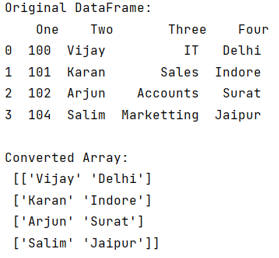# Convert Select Columns in Pandas Dataframe to NumPy Array

Given a Pandas DataFrame, we have to convert its selected columns to Numpy Array. By Pranit Sharma Last updated : September 24, 2023

Pandas is a special tool that allows us to perform complex manipulations of data effectively and efficiently. Inside pandas, we mostly deal with a dataset in the form of DataFrame. DataFrames are 2-dimensional data structures in pandas. DataFrames consist of rows, columns, and data.

## Converting DataFrame's Columns NumPy Array

To achieve this task, we will first create a DataFrame with multiple columns, let us say we have 4 columns like 'One', 'Two', 'Three', and 'Four'. We can check the type of this 2-dimensional table and we will get that it is a DataFrame.

To select some of the columns we will encapsulate all the required columns in a list and pass it as the index of DataFrame. For example, if we want columns 'Two' and 'Four' to be converted into a NumPy array, we will use the following code snippet.

```col_to_be_converted = df[["Two", "Four"]]
```

After this, we will use to_numpy() method which will allow us to convert out selected columns into a NumPy array we can also check the data type of this converted array and we will observe that it is of ndarray.

Let us understand with the help of an example,

## Python Program to Convert DataFrame's Columns to NumPy Array

```# Importing pandas package
import pandas as pd

# Creating a dictionary
d = {
'One':[100,101,102,104],
'Two':['Vijay','Karan','Arjun','Salim'],
'Three':['IT','Sales','Accounts','Marketting'],
'Four':['Delhi','Indore','Surat','Jaipur']
}

# Creating a DataFrame
df = pd.DataFrame(d)

# Display original DataFrame
print("Original DataFrame:\n",df,"\n")

# Converting some columns to numpy array
cols_to_be_selected = df[['Two','Four']].to_numpy()

# Display new array
print("Converted Array:\n",cols_to_be_selected)
```

### Output

The output of the above program is: